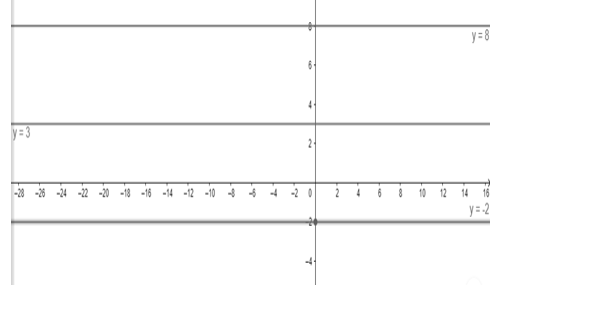# Find the equation of a line which is equidistant from the lines`
Question:

Find the equation of a line which is equidistant from the lines y = 8 and y = - 2.

Solution:

For the equation of line equidistant from both lines, we will find point through which line passes and is equidistant from both line.

As any point lying on $y=8$ line is $(0,8)$ and on $y=-2$ is $(0,-2)$, so mid - point is

$(\mathrm{x}, \mathrm{y})=\left(\frac{0+0}{2}, \frac{8-2}{2}\right)$

(x, y) = (0, 3)So, equation of line is y = 3.